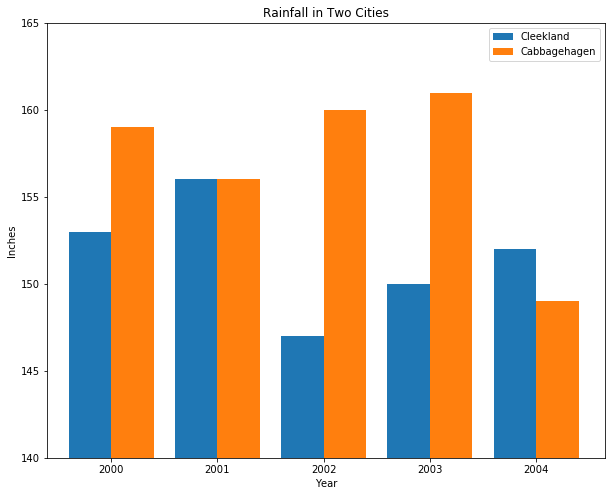List comprehensions are one of the easiest and most efficient ways to create lists in Python. This article will give you an overview of how list comprenhension works and show you several examples. If you would rather watch some video instruction, the following video will cover the same material. Happy comprehending!

List Comprehension

When doing data analysis, we often work with lists of numbers and need to modify and perform computations on them efficiently. Let’s say we are working with this list of temperatures, in Celsius, representing some results of a science experiment:

```temperatures = [-5, 29, 26, -7, 1, 18, 12, 31]
```

What if we found out that our lab thermometer was actually consistently reporting 20 degrees lower than it should have been? We want to add 20 to each temperature in the list. We could do this by hand and just write out a new list:

```temperatures_adjusted = [15, 49, 46, 13, 21, 38, 32, 51]
```

But that method is time-consuming and prone to errors. And what if our list was thousands of temperatures long? It wouldn’t be practical. It can be helpful to perform a function on all values of a list. Python can help us do this with list comprehensions. We can use this syntax to perform a list comprehension that would yield the same output:

```temperatures = [-5, 29, 26, -7, 1, 18, 12, 31]
temperatures_adjusted = [temp + 20 for temp in temperatures]
# temperatures_adjusted is now [15, 49, 46, 13, 21, 38, 32, 51]
```

This list comprehension:

1. takes an element in `temperatures`
2. names that element `temp`
3. stores the value of `temp + 20` in a new list called `temperatures_adjusted`
4. repeats steps 1-3 for all of the values in `temperatures`

Note that if we hadn’t done any mathematical operations on `temp`, the new list would be just a copy of `temperatures`:

```temperatures = [-5, 29, 26, -7, 1, 18, 12, 31]
temperatures_new = [temp for temp in temperatures]
# temperatures_new is now [-5, 29, 26, -7, 1, 18, 12, 31]
```

We can do more complicated mathematical operations like multiplication and division in our list comprehensions. This is how we would convert the `temperatures` list into Fahrenheit:

```temperatures = [-5, 29, 26, -7, 1, 18, 12, 31]
temperatures_F = [(9.0/5.0)*temp + 32 for temp in temperatures]
# temperatures_F is now [23.0, 84.2, 78.8, 19.4, 33.8, 64.4, 53.6, 87.8]
```

List comprehensions can be useful in many different scenarios. For example, consider having to create multiple lists of the x-values for a bar chart, where we are displaying values side-by-side like this:For this chart, we had two datasets that we wanted to plot at two different sets of x-values. The bars have a width of 0.8 (a standard for the graphing library Matplotlib), and we want the bars to be touching. We would want the first blue bar to be at x = 0.0, and the first orange bar to be at x=0.8, so that the bars are touching. The second blue bar would go at x=2.0, and the second orange bar at x=2.8, and so on.

Instead of calculating each one of these by hand, we can use a list comprehension:

```x_values_1 = [2*index for index in range(5)]
# [0.0, 2.0, 4.0, 6.0, 8.0]
x_values_2 = [2*index + 0.8 for index in range(5)]
# [0.8, 2.8, 4.8, 6.8, 8.8]
```

To calculate `x_values_2`, we went through the list `range(5)`, which is `[0, 1, 2, 3, 4]`. We picked out each element, called it `index`, and then calculated `2*index + 0.8`. The answer for each index was then stored in the `x_values_2` list. The x-tick labels (the ones that say 2000, 2001, 2002, etc) were placed at each midpoint of the two bars. How did we calculate those x-values? The midpoint of 0.0 and 0.8 is 0.4, so that’s where the first tick should be. The midpoint of 2.0 and 2.8 is 2.4, so that’s where the second tick should be. Using a list comprehension:

```x_values_midpoints = [(x1 + x2)/2.0 for (x1, x2) in zip(x_values_1, x_values_2)]
# [1.2, 3.2, 5.2, 7.2, 9.2]
```

To be able to go through two lists (`x_values_1` and `x_values_2`) in one list comprehension, we used the built-in Python function `zip`, which puts the elements of two lists together into one list. For example,

```zip([1, 2, 3], [4, 6, 8])
```

yields:

```[(1, 4), (2, 6), (3, 8)]
```

The list comprehension is a concise and powerful tool to modify Python lists in one line.